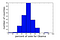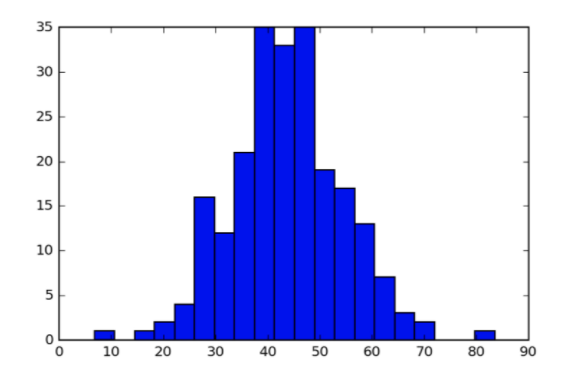# Statistical Thinking in Python (Part 1)

This article is about my lecture note from this course and some implementation of idea.

# Lecture note: Graphical exploratory data analysis

In this part of course, it introduces three commonly-used plot in data visualization:

## 1. HistogramThe data is from 2008 US swing state election results

By the histogram above we can easily tell that Barack Obama got less than 50 percent of vote of the majority counties in swing states.

However, there are two drawbacks while using histogram:

• Binning bias: the same data may be interpreted differently depending on choice of binsleft: number of bins = 10; right: number of bins = 20
• We can’t know the exact value of data from histogram.

To deal with the problems above, the swarm plot might be the possible candidate.

## 2. Swarm plot

The swarm plot above tells us that, in PA, OH, FL three states, Barack Obama got less than 50 percent of vote of the majority counties (the points below the black line), and we can know the value of each data.

However, when the number of data is large, you might be confused about the plot.

It’s hard to tell each point from others.

To deal with the problems above, ECDF(Empirical cumulative distribution function) is a good solution.

## 3. ECDF(Empirical cumulative distribution function)

To generate an ECDF, we need to sort our data first. The x-value of an ECDF is the quantity you are measuring, and the y-value is the fraction of data that have a value smaller than the corresponding x-value.

Also, we can plot several ECDFs on the same plot to compare.

By ECDF, we can easily realize the distribution of data. Hurray!

# My turn: idea implementation

I decided to use the EDA techniques above on the Titanic project I’m working on.

I would like to know if there is any relation between two features `Pclass` and `Fare`.

## Data preprocessing in 3 steps

Step 1: Import libraries and data(you can download the data here)

Step 2: Fill in the missing value of fare in testing data. (I use the mean fare of group with the same`Pclass` and `Embarked` to fill in, because I think these two features affect `Fare`the most）

Step3: Log transformation (Since the value of fare is wildly distributed, by doing transformation, we can observe the relation easier.)

## Histogram

Note that I choose the square root of number of data as the number of bin. The “square root rule” is a commonly-used rule of thumb for choosing number of bins.

And I let the number of bins of each histogram fixed, which can help us do the comparison more easily.

The histogram above shows us people in `Pclass_1` tend to pay more fare than people in `Pclass_2` and `Pclass_3`.

But what if I would like to know the exact value of each data? Let’s move on to the swarm plot.

## Swarm plot

Because now we can see the value of each data point, we have a sense that the average fare relation is `Pclass_1` > `Pclass_2` > `Pclass_3`.

Compare with histogram, it’s more clear and informative, isn’t it?

# ECDF

From ECDF above, we can randomly choose a y value to know the fare relation among `Pclass`.

For example, let’s try y = 0.5, which means that corresponding data point is the 50 quantile (a.k.a. median). The median of the Pclass_1 is the greatest.

And we can find that there is a positive relationship between `Pclass` and `Fare`.The passengers in Pclass_1 tend to pay more than that in Pclass_2 and Pclass_3.

What’s more, it gives the big picture of how fare data distributed!

## Add survival relationship

Because the goal of this project is to predict which passengers survived based on the given features, I try to combine the survival result with the two features mentioned above and plot the ECDF again.

The result is impressive. From the ECDF below, we know another information: within the same `Pclass`, the survived passengers tend to pay more than the dead passengers.

After the practice of the tools above, I know how powerful the ECDF is. I think I will use it quite often in the future:)

If you have any questions, feel free to leave a comment below:)

On my way to data scientist | Nature lover

## More from 潘彥銘

On my way to data scientist | Nature lover